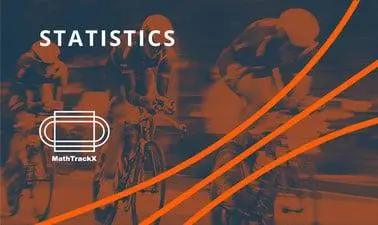There is 0 item now in your comparison listView a comparison list

# MathTrackX: Statistics

Price
Free
5,241 people completed this program

## Overview

This course is part six of the MathTrackX XSeries Program which has been designed to provide you with a solid foundation in mathematical fundamentals and how they can be applied in the real world.

This course will build on probability and random variable knowledge gained from previous courses in the MathTrackX XSeries with the study of statistical inference, one of the most important parts of statistics.

Guided by experts from the School of Mathematics and the Maths Learning Centre at the University of Adelaide, this course will cover random sampling, sample means and proportions, confidence intervals for sample means and proportions and one-sample tests of proportions and means.

Join us as we provide opportunities to develop your skills and confidence in applying mathematics to solve real world problems.

• The concept of a random sample, sources of bias in samples, and procedures to ensure randomness

• The concept of the sample proportion as a random variable

• The approximate normality of the distribution of proportions for large samples

• The concept of an interval estimates for a parameter associated with a random variable

• How to define the approximate margin of error for proportions.

## Authored by